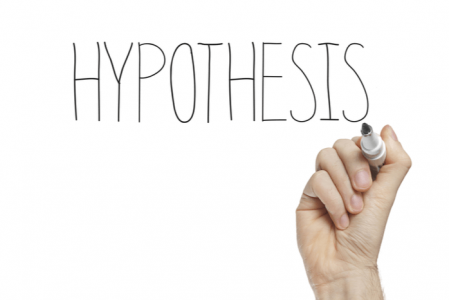# How to Write a Hypothesis in 5 Easy Steps

Discover tips and find info about What to Write About in a BlogOur experts from BookWormLab tried hard to make you inspired!

Our experts from BookWormLab tried hard to make you inspired!

Want to get unique help with writing?

Hire a WriterOften students confuse theory and hypothesis, speaking of a theory as something that needs proof. In science, a good theory is always based on a hypothesis, which has already been proved right many times. However, not all hypotheses lead to theories, as researchers may prove them to be right or wrong. At the end of the day, a hypothesis turns out to be a key methodological element of research…

What Is a Research Hypothesis?

In simple terms, a research hypothesis is a statement, in which the researcher assumes the existence of a relationship between several variables. It is an “if-then” statement, frequently talked about as an “educated guess”. A hypothesis is a predicted outcome of a study (or research). The task of the author of the hypothesis statement is to empirically prove or refute this statement.

Where Is a Hypothesis Placed in Research?

A research hypothesis is part of an introduction. It is often placed after the description of the goal and objectives and before the chapter dedicated to the research methods.

A scientific hypothesis in the introduction should only be noted, not solved. Therefore, it will be necessary to prove or disprove it after the performed research. A statement of a hypothesis should occupy not more than a paragraph of text. Therefore, it is important to correctly write a hypothesis so that it is understandable and logical to the readers (fellow researchers or the public).

How to Write a Hypothesis

It is necessary to consider a hypothesis according to two criteria:

1. It is based on a scientific problem and should be understandable to the researcher
2. A hypothesis can be tested using scientific methods

One can say that a hypothesis is an assumption of a solution to a scientific problem or its mitigation. At the end of a thesis or coursework, a conclusion is made whether the hypothesis is true or not.

That is, using methods such as analysis, synthesis, or comparison, the researcher conducts a study and establishes whether the proposed hypothesis is true or false.

It is necessary to use your own guesses for the solution that appeared when you got acquainted with the problem. If there are no ideas, then it is recommended to write the theoretical and practical part first. This will help you better understand the problem under study and the subject of research.

Templates to Help Formulate a Hypothesis

Use the following templates to express your research hypothesis:

• You can expect something to improve thanks to …
• It can be assumed that something will be successful if …
• It can be assumed that if something happens, then …
• It is assumed that the improvement will come after the application …

Types of Hypotheses

By its nature, a hypothesis can be revolutionary (putting forward something fundamentally new) or a modification of known laws, based on the assumption that some laws exist in areas where their effect has not yet been revealed.

According to the logical structure, hypotheses can be linear, when one assumption is put forward and tested, or branched when several assumptions need to be tested.

5 Easy Steps to Write a Hypothesis

Finally, here are 5 logical and easy steps to help you write a research hypothesis:

1. Understand your topic. Without knowing and understanding the research topic, you won’t be able to formulate a good hypothesis. Make sure you understand your topic well and have enough motivation to dig into the previous research to formulate a unique hypothesis.
2. Make an educated guess. A hypothesis doesn’t come out of nowhere. Its appearance is preceded by various guesses that do not represent a hypothesis as such. A guess can be called a hypothesis if it includes a rationale based on proven propositions.
3. Connect your guess with the research topic. It must be remembered that the formulation of a hypothesis is carried out on the basis of the problem under study. A brilliantly formulated hypothesis meets such requirements as adequacy to the research question, absence of conflict between new and old knowledge, and availability for verification. In addition, the prediction you make in your hypothesis must be interesting and topical, not reduced to trivial facts.
4. Define variables. Variables are important to prove or refute your hypothesis. There are dependent and independent variables. Make sure that you can influence the independent variables in order to conduct your experiments/research/study.
5. Phrase your hypothesis. The last but very significant step is to phrase your hypothesis. Remember the simple recipe “if-then” to make the hypothesis clear and easy to understand not only to your research fellows but to the public as well.